# nLab intersecting D-brane model

Contents

## Phenomenology

#### Fields and quanta

field (physics)

standard model of particle physics

force field gauge bosons

scalar bosons

flavors of fundamental fermions in the
standard model of particle physics:
generation of fermions1st generation2nd generation3d generation
quarks ($q$)
up-typeup quark ($u$)charm quark ($c$)top quark ($t$)
down-typedown quark ($d$)strange quark ($s$)bottom quark ($b$)
leptons
chargedelectronmuontauon
neutralelectron neutrinomuon neutrinotau neutrino
bound states:
mesonslight mesons:
pion ($u d$)
ρ-meson ($u d$)
ω-meson ($u d$)
f1-meson
a1-meson
strange-mesons:
ϕ-meson ($s \bar s$),
kaon, K*-meson ($u s$, $d s$)
eta-meson ($u u + d d + s s$)

charmed heavy mesons:
D-meson ($u c$, $d c$, $s c$)
J/ψ-meson ($c \bar c$)
bottom heavy mesons:
B-meson ($q b$)
ϒ-meson ($b \bar b$)
baryonsnucleons:
proton $(u u d)$
neutron $(u d d)$

(also: antiparticles)

effective particles

hadron (bound states of the above quarks)

solitons

minimally extended supersymmetric standard model

superpartners

bosinos:

dark matter candidates

Exotica

auxiliary fields

# Contents

## Idea

In string phenomenology for type IIA string theory, quasi-realistic models (i.e. close to the standard model of particle physics, or an MSSM) may be obtained by KK-compactifications from the 10-dimensions down to 4d with configurations of D6-branes which fill all of 4d spacetime and intersect in a certain way in the 6-dimensional fiber space.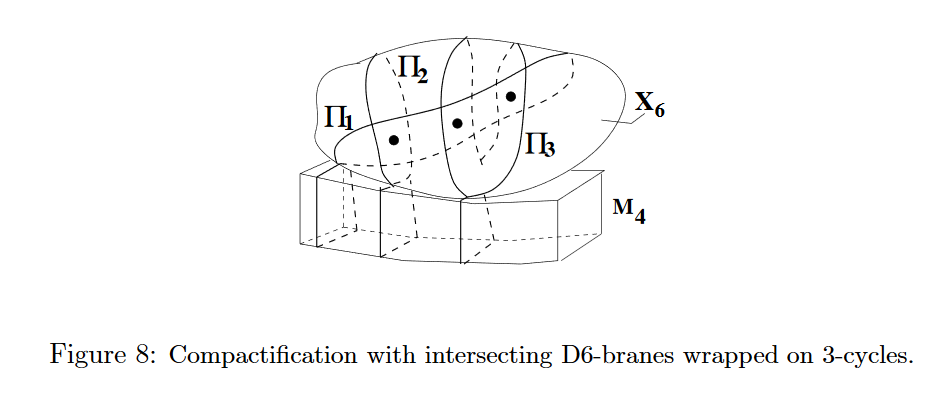graphics grabbed from Uranga 12a, p. 12

The Chan-Paton gauge field on the D6-branes yields the gauge fields in 4d, and the precise intersection pattern determines the effective fundamental particle content in 4d.

See also at string phenomenology the section Models in type II with intersecting branes.

## Bottom-up and Top-down approaches

One distinguishes bottom-up and top-down model building strategies: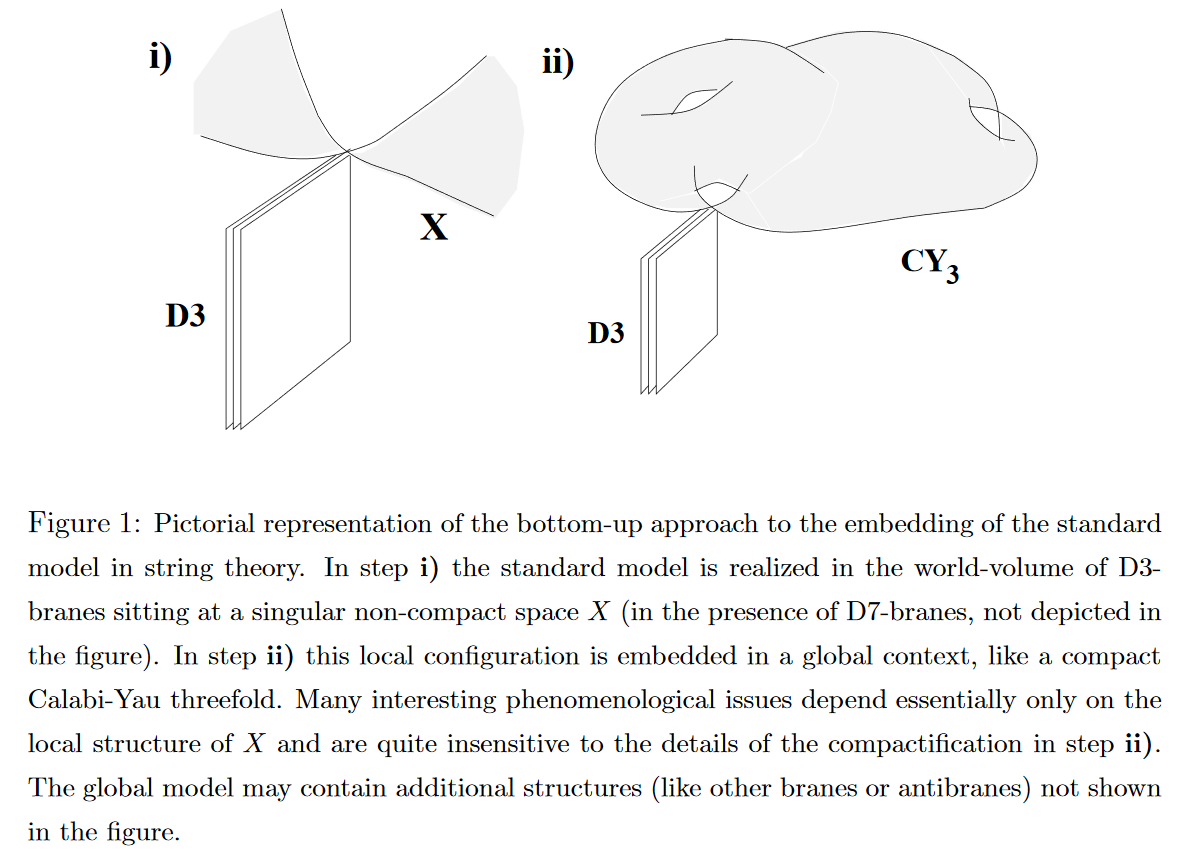snippet grabbed from Aldazabal-Ibáñez-Quevedo-Uranga 00

## Properties

### Chiral fermions

One of the most striking special properties of the standard model of particle physics is that its content of fermionic fundamental particles is “chiral”, in that the left and right Weyl spinor-components of the would-be Dirac spinor-representation of the quarks, electrons and neutrinos couple differently to the gauge fields (for review see e.g. Ibanez-Uranga 12, section 1.1).

The observation that such chiral fermions do indeed appear when D6-branes intersect at an angle on an $\mathbb{R}^{3,1}$ is due to (Berkooz-Douglas-Leigh 96), see also (AFIRU 00, section 4, BCLS 05, section 2.3). For review with an eye towards RR-field tadpole cancellation on toroidal orientifolds see also Forste-Honecker-Schreyer 01, Honecker 01, Sec. 2, Honecker 02.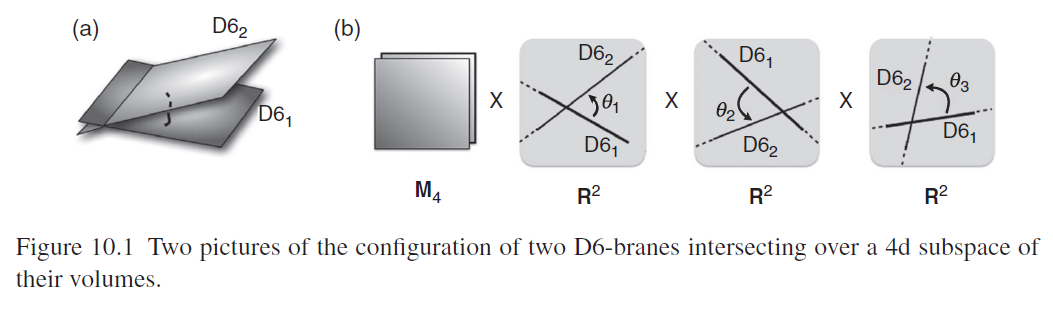graphics grabbed from Ibáñez-Uranga 12

Vague review is in (Ibáñez-Uranga 12, section 10.2.1, Uranga 12a, section 2.3).

The lift of this situation to M-theory on G2-manifolds is discussed in (Berglund-Brandhuber 02, Bourjaily-Espahbodi 08).

### Generations of fermions

While (presently) intersecting D-brane models don’t explain why there are precisely 3 generations of fundamental particles in the standard model of particle physics, they do have the property that generically any such model does feature several generations of fundamental particles.

The reason is that in these models there is one copy of a set of fundamental particles at each intersection point of two 3-manifolds (the internal part of the D6-branes) in a compact 6-dimensional space, and generically these intersection numbers are greater than one and hence induce a finite number of generations (BCLS 05, section 2.3, Ibanez-Uranga 12, p. 307, Uranga 12a, p. 12):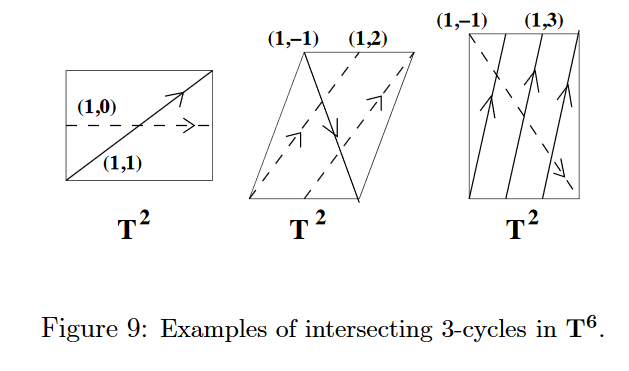graphics grabbed from Uranga 12a, p. 13

### Yukawa couplings

In intersecting D-brane models Yukawa couplings are encoded by worldsheet instantons of open strings stretching between the intersecting D-branes (see Marchesano 03, Section 7.5). Mathematically this is encoded by derived hom-spaces in a Fukaya category (see Marchesano 03, Section 7.5).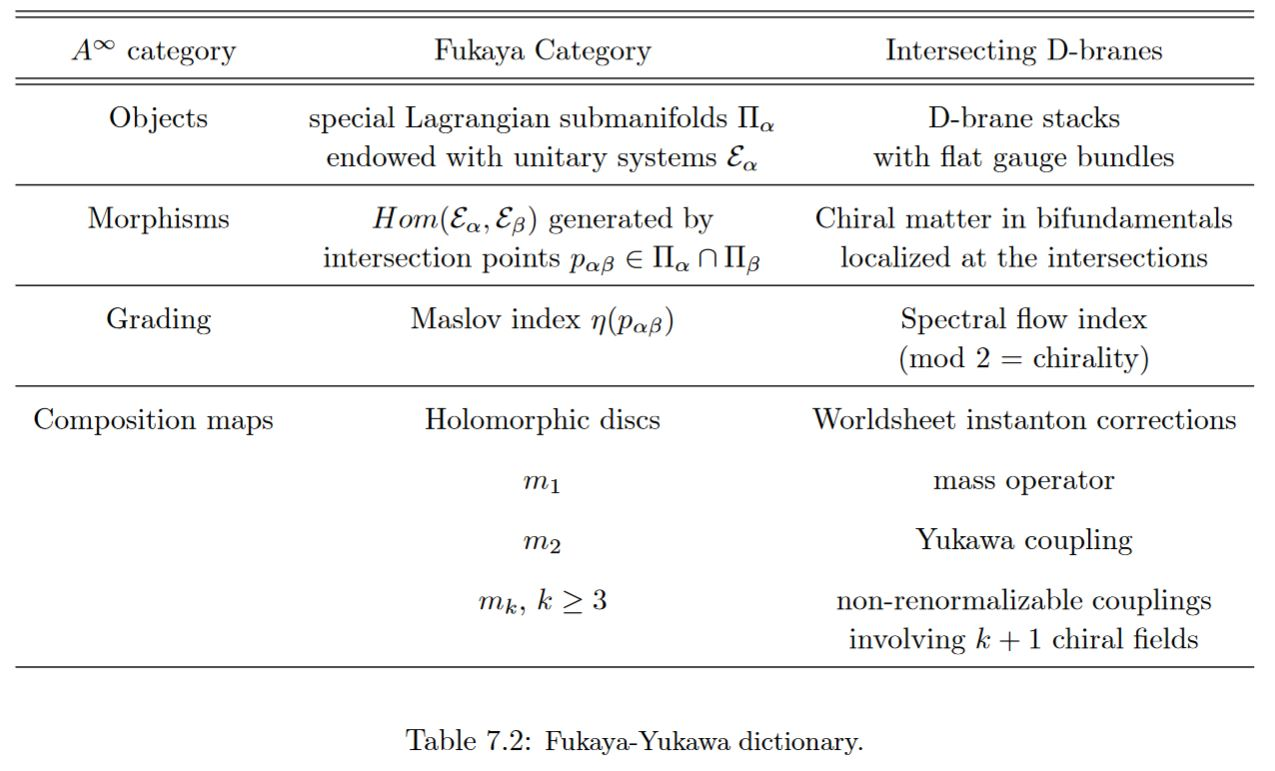table grabbed from Marchesano 03

Realistic Yukawa couplings and fermion masses in an MSSM Pati-Salam GUT model with 3 generations of fermions realized on intersecting D6-branes KK-compactified on a toroidal orbifold $T^6\sslash (\mathbb{Z}_2 \times \mathbb{Z}_2)$ are claimed in in Mayes 19, Gemill-Howington-Mayes 19, based on Chen-Li-Mayes-Nanopoulos 07a, Chen-Li-Mayes-Nanopoulos 07b.

### Higgs mechanism

The Higgs mechanism naturally arises in intersecting D-brane models: The Higgs field appears here as the scalar field that witnesses in perturbation theory the process of brane recombination at the intersection locus of the D-branes (Cremades-Ibanez-Marchesano 02, section 7):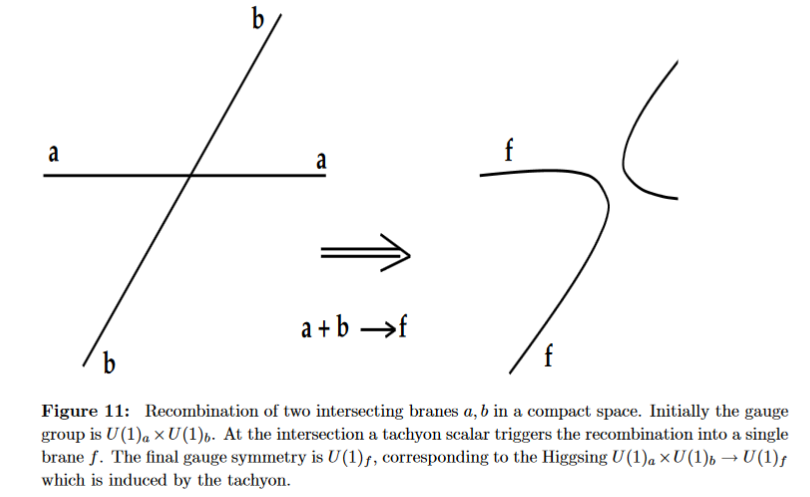Further developments in Ibanez-Marchesano-Rabadan 01, Hebecker-Knochel-Weigand 13 and specifically via string field theory in HINSS 18.

For review see Ibanez-Uranga 12, fig 10.2: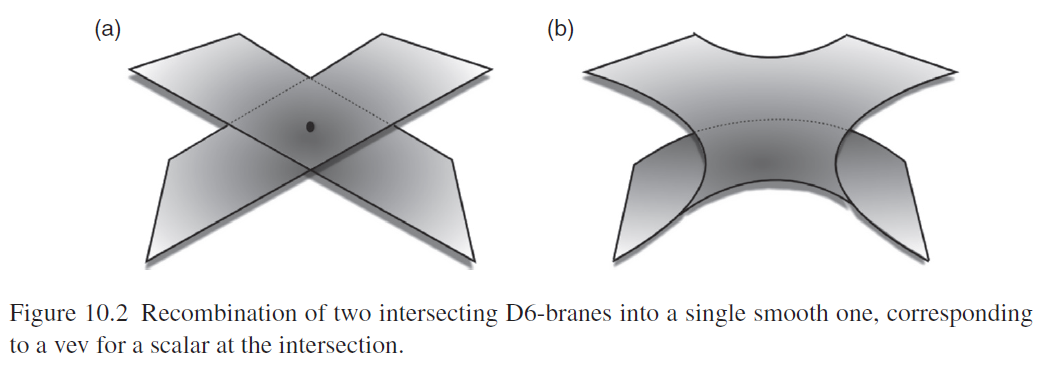Consistent intersecting D-brane models have to be in type I string theory, or generally in type II string theory with orientifold backgrounds, to achieve RR-field tadpole cancellation.

This is a key consistency condition in intersecting D-brane model building (e.g. BCLS 05, section 2.4, Ibanez-Uranga 12, section 4.4)

RR-field tadpole cancellation conditions for D-branes wrapped on toroidal orientifolds
in terms of their D-brane charge $V \in K_G(\ast) = R_G$ in equivariant K-theory = representation ring
(here $\mathbf{n}_{reg} = k[G/1]$ denotes the regular representation of dimension $n =$ ord(G))

single D-brane species
on toroidal orientifold
local/twisted
global/untwisted
comment
D5-branes
transv. to $\mathbb{T}^{\mathbf{4}_{\mathbb{H}}}\!\sslash\! \mathbb{Z}_{2}$
$V = N \cdot \mathbf{2}_{reg}$
(Buchel-Shiu-Tye 99 (19))
$V = 16 \cdot \mathbf{2}_{reg}$
(Buchel-Shiu-Tye 99 (18))
following
Gimon-Polchinski 96,
Gimon-Johnson 96
D5-branes
transv. to $\mathbb{T}^{\mathbf{4}_{\mathbb{H}}} \!\sslash\! \mathbb{Z}_{4}$
$V = N \cdot \mathbf{4}_{reg}$
(Buchel-Shiu-Tye 99 (19))
$V = 8 \cdot \mathbf{4}_{reg}$
(Buchel-Shiu-Tye 99 (18))
following
Gimon-Polchinski 96,
Gimon-Johnson 96
D4-branes
transv. to $\mathbb{T}^{\mathbf{1}_{\mathrm{triv}} + \mathbf{4}_{\mathbb{H}}} \!\sslash\! \mathbb{Z}_k$
$V = N \cdot \mathbf{k}_{reg}$
(AFIRU 00a, 4.2.1)
D4-branes
transv. to $\mathbb{T}^{\mathbf{1}_{\mathrm{triv}} + \mathbf{4}_{\mathbb{H}}} \!\sslash\! \mathbb{Z}_3$
$V = N \cdot \mathbf{3}_{reg}$
(AFIRU 00b, (7.2))
$V = 4 \cdot \mathbf{3}_{reg} + 4 \cdot \mathbf{1}_{triv}$
(Kataoka-Shimojo 01, (14)-(17)
D8-branes
on $\mathbb{T}^{\mathbf{1}_{\mathrm{triv}} + \mathbf{4}_{\mathbb{H}}} \!\sslash\! \mathbb{Z}_3$
$V = N \cdot \mathbf{3}_{reg}$
$V = 4 \cdot \mathbf{3}_{reg} + 4 \cdot \mathbf{1}_{triv}$
(Honecker 01, 4,
Honecker 02a, (25) ∧ (28) ⇔ (29),
Honecker 02b, (3.19)-(3.27))
equivalent to D4 case by T-duality:
Honecker 01, p. 2,
Honecker 02a, 6,
Honecker 02b, p. 15
review in:
Marchesano 03, Sec. 4
D6-branes
on $\mathbb{T}^6 \!\sslash\! \mathbb{Z}_4$
$V = 8 \cdot \mathbf{4}_{reg}$
(Ishihara-Kataoka-Sato 99, (4.16))
D3-branes
on $\mathbb{T}^{\mathbf{4}_{\mathbb{H}}} \!\sslash\! \mathbb{Z}_k$
$V = N \cdot \mathbf{k}_{reg}$
(Feng-He-Karch-Uranga 01, (25))
D7-branes
on $\mathbb{T}^{\mathbf{4}_{\mathbb{H}}} \!\sslash\! \mathbb{Z}_k$
$V = N \cdot \mathbf{k}_{reg}$
(Feng-He-Karch-Uranga 01, (5), (6))

### Intersections of D6s with D8/O8s

A black D6-brane may end on a black NS5-brane, and in fact each brane NS5-brane at a non-trivial ADE singularity has to be the junction of two black D6-branes. For details see at D6-branes ending on NS5-branes.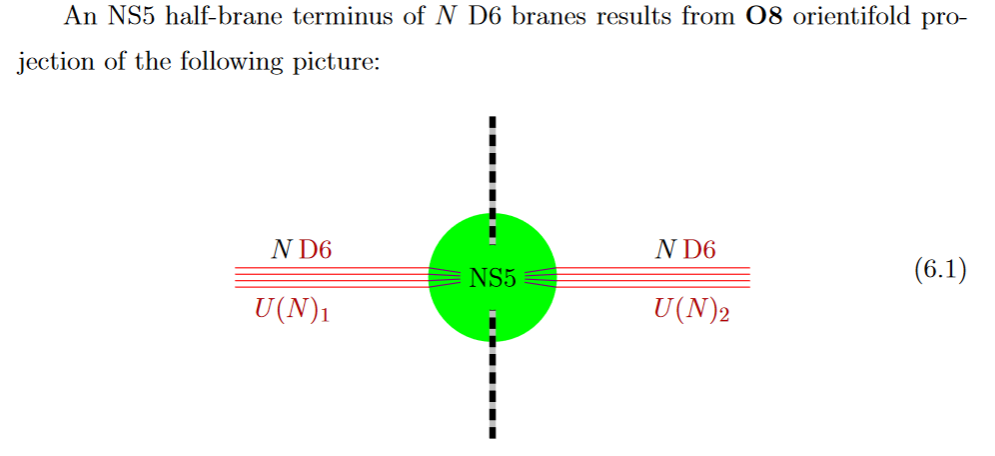from GKSTY 02

If in addition the black NS5-brane sits at an O8-plane, hence at the orientifold fixed point-locus (see above), then in the ordinary $\mathbb{Z}/2$-quotient it appears as a “half-brane” with only one copy of D6-branes ending on it: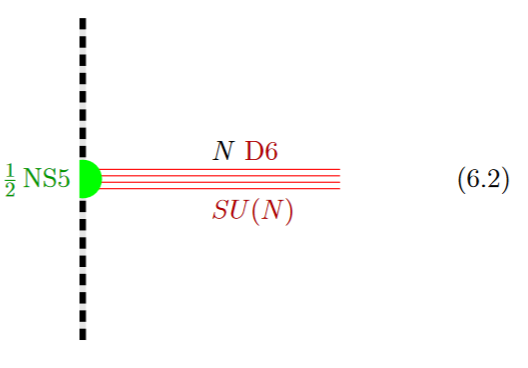from GKSTY 02

(In Hanany-Zaffaroni 99 this is interpreted in terms of the 't Hooft-Polyakov monopole.)

The lift to M-theory of this situation is an M5-brane intersecting an M9-brane: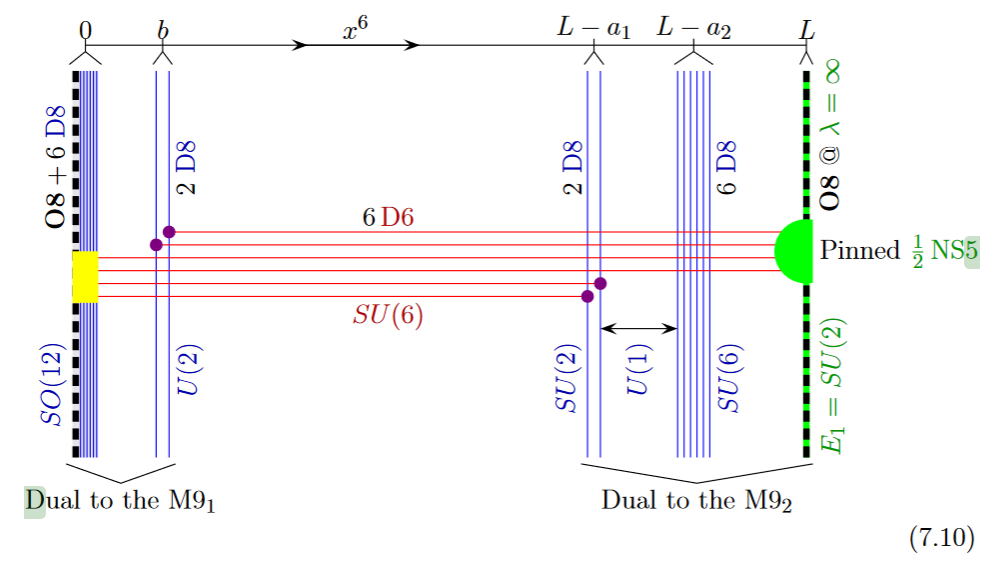from GKSTY 02

Alternatively, the O8-plane may intersect the black D6-branes away from the black NS5-brane: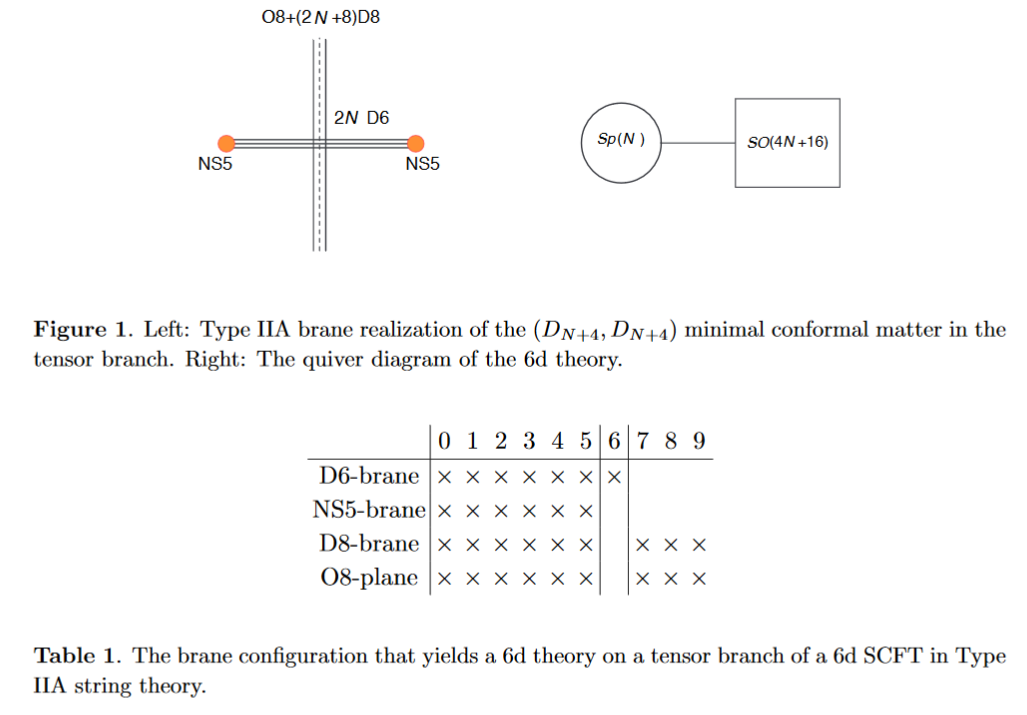from HKLY 15

In general, some of the NS5 sit away from the O8-plane, while some sit on top of it: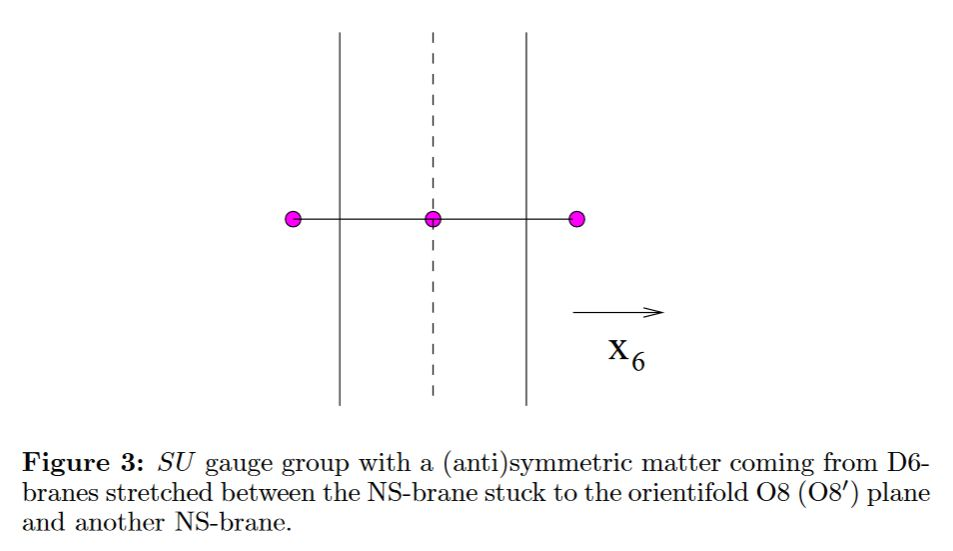### Relation to M-theory on $G_2$-manifolds

Lift to M-theory on G2-manifolds (e.g. G2-MSSM): see references below

$\,$

### Cosmology and Holography

Since the near horizon geometry of BPS black branes is conformal to the Cartesian product of anti de Sitter spaces with the unit $n$-sphere around the brane, the cosmology of intersecting D-brane models realizes the observable universe on the asymptotic boundary of an approximately anti de Sitter spacetime (see for instance Kaloper 04, Flachi-Minamitsuji 09). The basic structure is hence that of Randall-Sundrum models, but details differ, such as notably in warped throat geometries, see Uranga 05, section 18.

These warped throat models go back to Klebanov-Strassler 00 which discusses aspects of confinement in Yang-Mills theory on conincident ordinary and fractional D3-branes at the singularity of a warped conifold. See also Klebanov-Witten 98.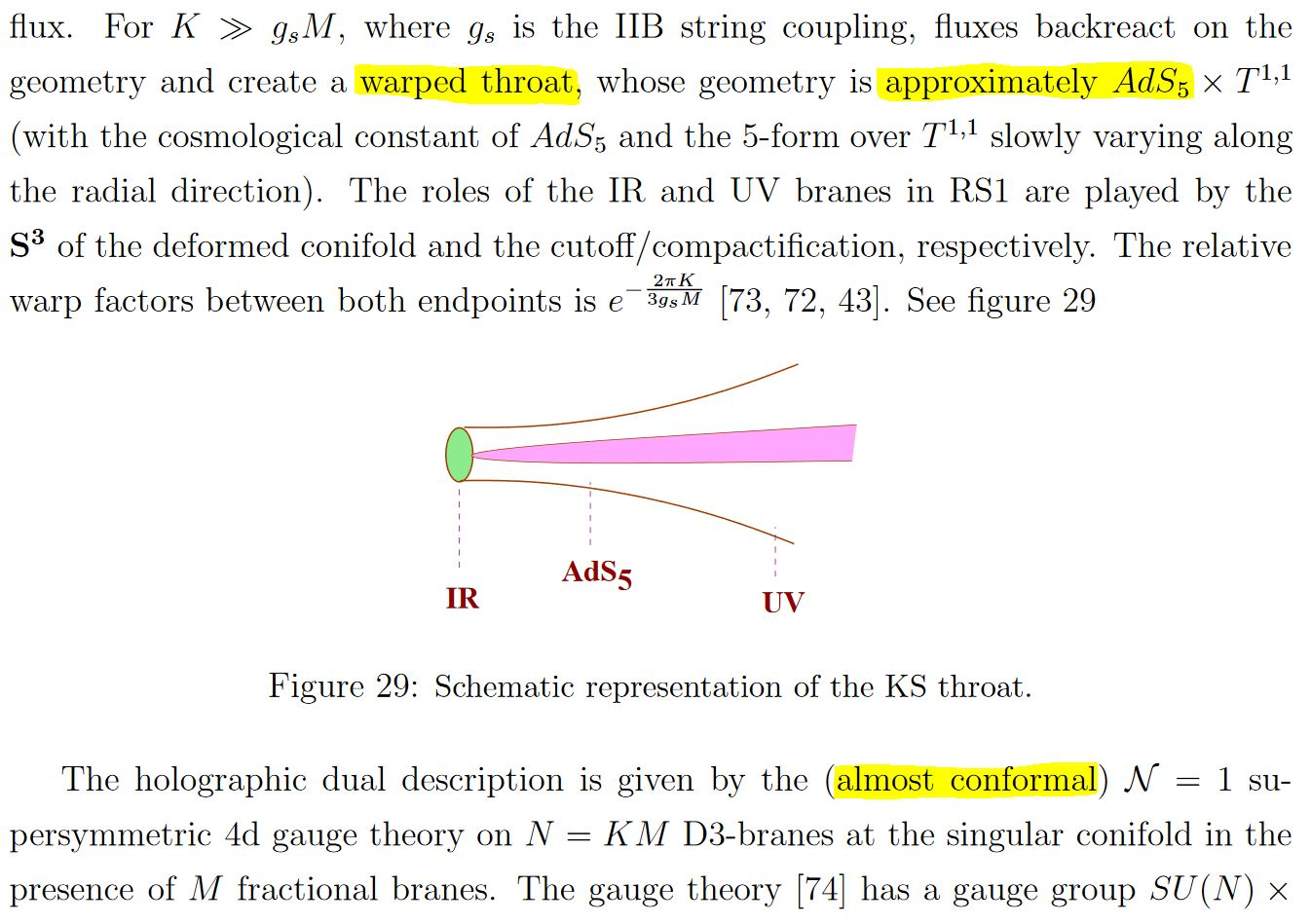snippet grabbed from Uranga 05, section 18

here: “RS”=Randall-Sundrum model; “KS”=Klebanov-Strassler 00

In particular this means that AdS-CFT duality applies in some approximation to intersecting D-brane models (e.g. Soda 10, GHMO 16), thus allowing to compute, to some approximation, non-perturbative effects in the Yang-Mills theory on the intersecting branes in terms of gravity on the ambient warped throat $\sim$ AdS (Klebanov-Strassler 00, section 6)

Such approximate versions of AdS-CFT for gauge theories realized on intersecting D-branes are used for instance to estimate non-perturbative effects in QCD, such as the shear viscosity of the quark-gluon plasma (see the references there). See at AdS-QCD correspondence for more on this.

$\,$

### Computer scan of Gepner-model compactifications

Discussion of string phenomenology of intersecting D-brane models KK-compactified with non-geometric fibers such that the would-be string sigma-models with these target spaces are in fact Gepner models (in the sense of Spectral Standard Model and String Compactifications) is in (Dijkstra-Huiszoon-Schellekens 04a, Dijkstra-Huiszoon-Schellekens 04b):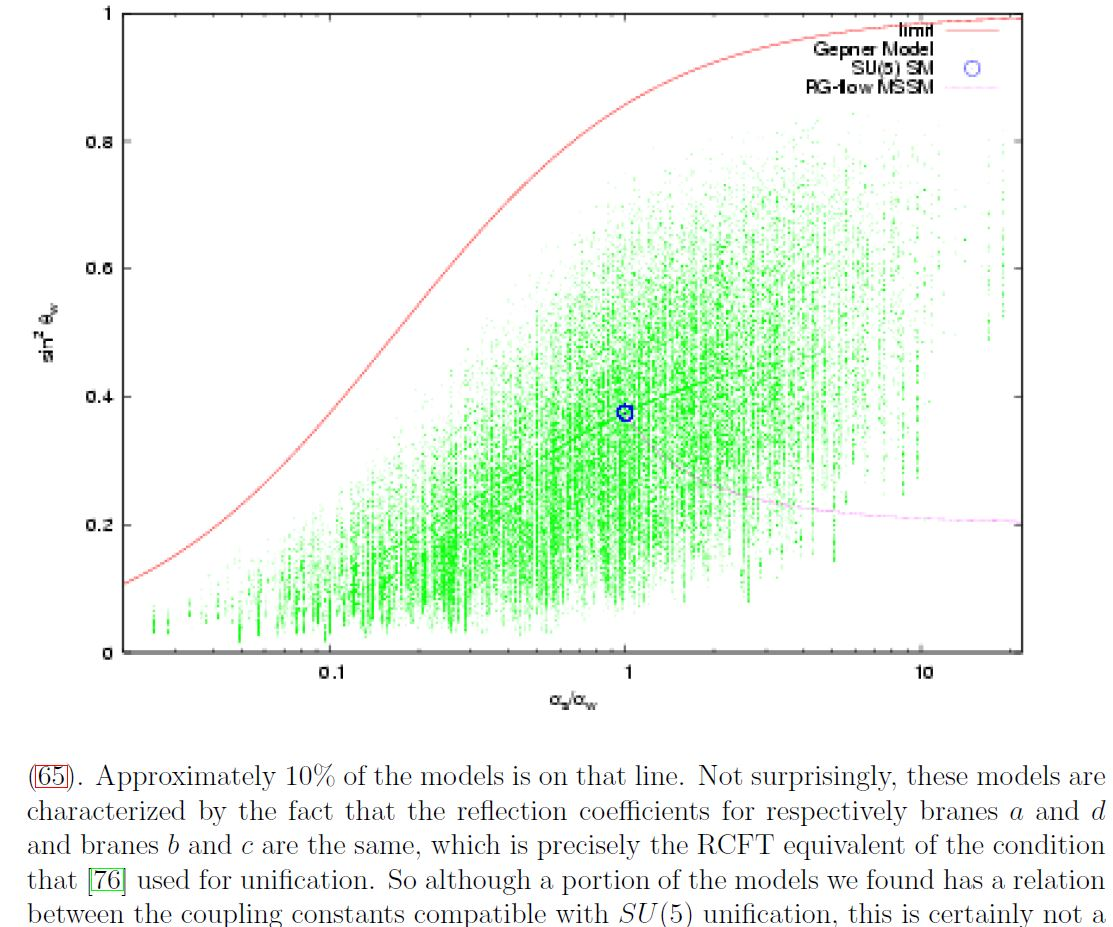A plot of standard model-like coupling constants in a computer scan of Gepner model-KK-compactification of intersecting D-brane models according to Dijkstra-Huiszoon-Schellekens 04b.

The blue dot indicates the couplings in $SU(5)$-GUT theory. The faint lines are NOT drawn by hand, but reflect increased density of Gepner models as seen by the computer scan.

## Examples

### General

brane intersections/bound states/wrapped branes

S-duality$\,$bound states:

intersecting$\,$M-branes:

### Intersecting D6-brane models

(…)

• S. Ishihara, H. Kataoka, Hikaru Sato, $D=4$, $N=1$, Type IIA Orientifolds, Phys. Rev. D60 (1999) 126005 (arXiv:hep-th/9908017)

(…)

### Intersecting D4-brane models

Only D4-branes:

• D. Bailin, G. V. Kraniotis, A. Love, Standard-like models from intersecting D4-branes, Phys. Lett. B530 (2002) 202-209 (arXiv:hep-th/0108131)

• H. Kataoka, M. Shimojo, $SU(3) \times SU(2) \times U(1)$ Chiral Models from Intersecting D4-/D5-branes, Progress of Theoretical Physics, Volume 107, Issue 6, June 2002, Pages 1291–1296 (arXiv:hep-th/0112247, doi:10.1143/PTP.107.1291)

• D. Bailin, Standard-like models from D-branes, J Phys (2003) 60: 199 (arXiv:hep-th/0210227)

• D. Bailin, G. V. Kraniotis, A. Love, New Standard-like Models from Intersecting D4-Branes, Phys. Lett. B547 (2002) 43-50 (arXiv:hep-th/0208103)

give the Witten-Sakai-Sugimoto model for holographic QCD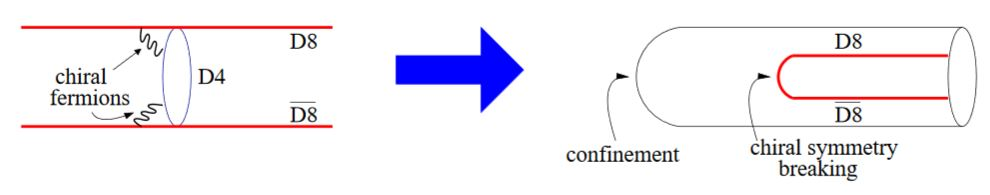graphics grabbed from Erlich 09, section 1.1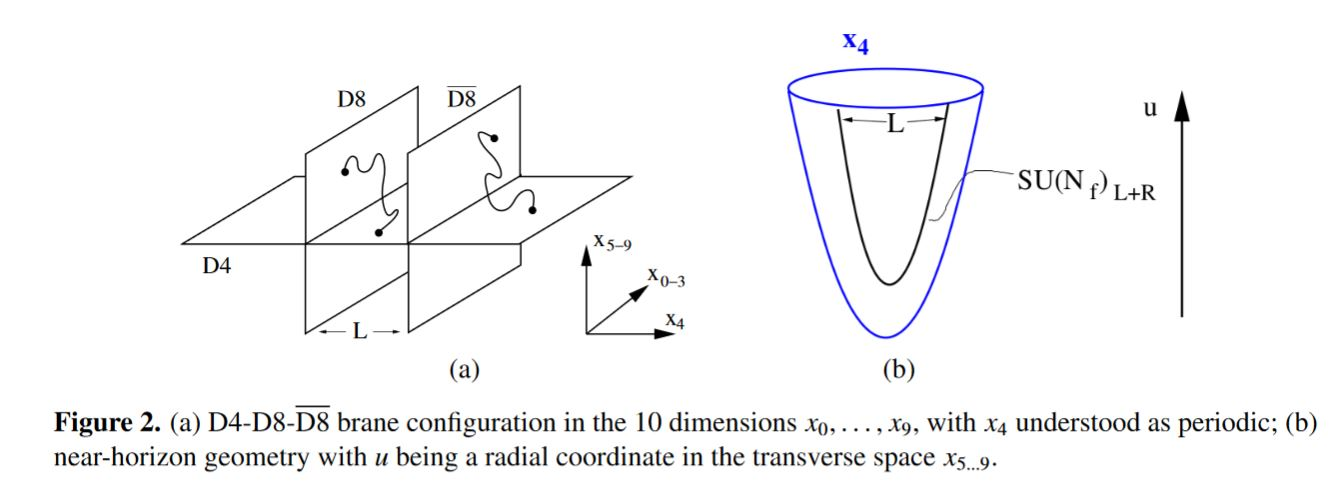graphics grabbed from Rebhan 14

(…)

### General

The bottom-up approach to intersecting D-brane model building was initiated in

The observation that chiral fermions appear when D6-branes intersect at an angle is due to

development for toroidal orientifolds is due to

Review includes

A textbook account is

Some chapters of which appear separately:

for D6-branes:

for D7-branes:

Discussion of the Higgs mechanism in intersecting D-brane models:

• G. Aldazabal, S. Franco, Luis Ibáñez, R. Rabadan, A. M. Uranga, $D=4$ Chiral String Compactifications from Intersecting Branes, J.Math.Phys.42:3103-3126, 2001 (arXiv:hep-th/0011073)

• Ralph Blumenhagen, Volker Braun, Boris Kors, Dieter Lüst, The Standard Model on the Quintic, Summary of Talks at SUSY02, 1st Intl. Conference on String Phenomenology in Oxford, Strings 2002 and 35th Ahrenshoop Symposium. (arXiv:hep-th/0210083)

• Dieter Lüst, Intersecting Brane Worlds – A Path to the Standard Model?, Class. Quant. Grav.21 : S1399-1424, 2004 (arXiv:hep-th/0401156)

• Ching-Ming Chen, Tianjun Li, Dimitri V. Nanopoulos, Standard-Like Model Building on Type II Orientifolds, Nucl.Phys.B732:224-242,2006 (arXiv:hep-th/0509059)

• Angel Uranga, The standard model from D-branes in string theory, talk at Padova, January 2008 (pdf)

• Matthew J. Dolan, Sven Krippendorf, Fernando Quevedo, Towards a Systematic Construction of Realistic D-brane Models on a del Pezzo Singularity, JHEP 1110 (2011) 024 (arXiv:1106.6039)

• Yuta Hamada, Tatsuo Kobayashi, Shohei Uemura, Standard Model-like D-brane models and gauge couplings, Nuclear Physics B Volume 897, August 2015, Pages 563-582 (arXiv:1409.2740)

• Jill Ecker, Gabriele Honecker, Wieland Staessens, D6-Brane Model Building on $\mathbb{Z}_2 \times \mathbb{Z}_6$: MSSM-like and Left-Right Symmetric Models, Nuclear Physics B Volume 901, December 2015, Pages 139-215, (arXiv:1509.00048)

Intersection with O8-planes/D8-branes (M-theory on S1/G_HW times H/G_ADE) is discussed in

### The landscape of models

On the landscape of intersecting D-brane models:

Computer scan of Gepner model-KK-compactifications of intersecting D-brane models:

Computer scan of toroidal orbifold-KK compactifications is discussed in

### Detailed realistic models

Realistic Yukawa couplings and fermion masses in an MSSM Pati-Salam GUT model with 3 generations of fermions realized on intersecting D6-branes KK-compactified on a toroidal orbifold $T^6\sslash (\mathbb{Z}_2 \times \mathbb{Z}_2)$ are claimed in

### Lift to M-theory

Lift of intersecting D-brane models to M-theory on G2-manifolds with ADE-singularities is discussed in the following articles

### Cosmology

Discussion for cosmology of intersecting D-brane models (ambient $\sim$ anti de Sitter spacetimes with the $\sim$ conformal intersecting branes at the asymptotic boundary) includes the following (see also at Randall-Sundrum model):

• Igor Klebanov, Matthew Strassler, Supergravity and a Confining Gauge Theory: Duality Cascades and $\chi^{SB}$-Resolution of Naked Singularities, JHEP 0008:052, 2000 (arXiv:hep-th/0007191)

• Igor Klebanov, Edward Witten, Superconformal Field Theory on Threebranes at a Calabi-Yau Singularity, Nucl.Phys.B536:199-218, 1998 (arXiv:hep-th/9807080)

• Luis Anchordoqui, Jose Edelstein, Carlos Nunez, Santiago Perez Bergliaffa, Martin Schvellinger, Marta Trobo, Fabio Zyserman, Brane Worlds, String Cosmology, and AdS/CFT, Phys. Rev. D64:084027, 2001 (arXiv:hep-th/0106127)

• Nemanja Kaloper, Origami World, JHEP 0405 (2004) 061 (arXiv:hep-th/0403208)

• Angel Uranga, section 18 of TASI lectures on String Compactification, Model Building, and Fluxes, 2005 (pdf, cern:933469)

• Antonino Flachi, Masato Minamitsuji, Field localization on a brane intersection in anti-de Sitter spacetime, Phys.Rev.D79:104021, 2009 (arXiv:0903.0133)

• Jiro Soda, AdS/CFT on the brane, Lect.Notes Phys.828:235-270, 2011 (arXiv:1001.1011)

• Gianluca Grignani, Troels Harmark, Andrea Marini, Marta Orselli, The Born-Infeld/Gravity Correspondence, Phys. Rev. D 94, 066009 (2016) (arXiv:1602.01640)

Survey is in

• Antonio Padilla, Braneworld Cosmology and Holography (arxiv:hep-th/0210217)

• Shunsuke Teraguchi, around slide 21 of: String theory and its relation to particle physics, 2007 (pdf)

Discussion of 4d de Sitter spacetime cosmology models on brane worlds in $\sim$ AdS bulk spacetimes:

### Experimental tests

Discussion of observational/experimental test of brane world models:

by analysis of the event horizon of the black hole in the center of the galaxy Messier 87:

• Indrani Banerjee, Sumanta Chakraborty, Soumitra SenGupta, Silhouette of M87${}^*$: A new window to peek into the world of hidden dimensions (arXiv:1909.09385)